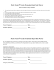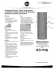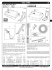GOVIND VIDYALAYA

Transcription

GOVIND VIDYALAYA
GOVIND VIDYALAYA
TAMULIA
Daily Practice Paper (DPP)
Subject-Mathematics
Practice Test
Test Module
Assignment
Practical Questions
Project
PRACTICE PAPER ON APPLICATION OF DERIVATIVE
[4 marks question]
1. The two equal side of on isosceles ∆ with fixed base b are decreasing at the rate of
3cm/s. How fast is the area decreasing when the two equal sides are equal to the base?
2. A men of height 2m walks at a uniform speed of 5km/h away from a lamp, past which is
6m high. Find the rate at which the lengths of his shadow increase.
3. A water tank has the shape of an inverted right circular cone with its axis vertical and
vertex lower most. Its semi vertical angle is
tan - 1 (0.5). water is poured into it at a
constant rate of 5cm 3/hr. Find the rate at which the level of the water is rising at the
instant when the depth of water in the tank is 4m.
4. Find the interval in which the function given by
F(x) =
x4 -
x3 - 3x2 +
x+ 1
is
(a) Strictly increasing (b) Strictly decreasing
5.Show that f(x) = tan -1 (sinx +cosx) is always an increasing function in( 0 , ).
6. For the curve y = 4x3 - 2x5 , find all the point at which the tangent passes through the
origin.
7. Prove that the curves x = y2 and xy = k cut at right angle if
8k2 = 1
[6 marks question]
8. Find the maximum area of an isosceles ∆ inscribed in the ellipse
+
= 1 with its
vertex at one end of the major axis.
9. A tank with rectangular base and rectangular sides, open at the top is to be constructe so
that its depth is 2m and volume is 8m3. If building of tank costs Rs 70 per sq. metres for the
base and Rs 45 per sq. metres for sides what is the cost of least expansive tank?
10. The sum of the perimeter of a circle and square is k, where K is some constant. Prove
that the sum of their area is least when the side of square is double the radius of circle.
11. A window is the form of a rectangle surmounted by a semi circular opening the total
perimeter of the window is 10m. Find the dimensions of the window to admit maximum
light through the whole opening.
12. A point on the hypotenuse of a triangle is at distance a and b from the sides of the
triangle. Show that the minimum length of the hypotenuse is (
+
)3/2
Designed & Developed By:
Name: Arbind Chakraborthy
Subject: Mathematics
Designation: HOD-Mathematics
Govind Vidyalaya, Tamulia

Dig Flyer - Terraquest Environmental Consultants, P.C.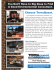preview your owner`s manual how to fill your water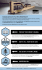Eagle Airfield - Used Equipment Data Sheet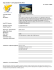to see more about our Social Think Tank Program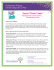TEST OR INSPECTION ITEM FREQUENCY REQUIREMENTS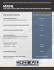RV Camping Checklist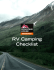Untitled - utahreefs.com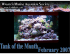Minneriya National Park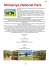descaling agent - Tensens Cleaning Supplies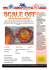hearts aflutter tank top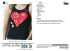Tanks 101 Course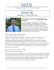Conference Agenda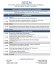www.koldkatcher.com Industrial Heat Trace Systems HEATED FUEL GAS THIEF HATCH HEATERS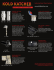Dunk Tank Release Form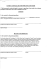RootsScrub E 4550 - Roots Multiclean Ltd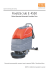ProSolutions Tank Cleaner MSDS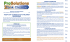LEVELWATCH BLE-C - NAVIS Anemometers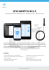Stuck thermostat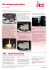todays-liquid-tank-codes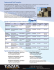Dunk TankSlip-n-SlideWaiver 15